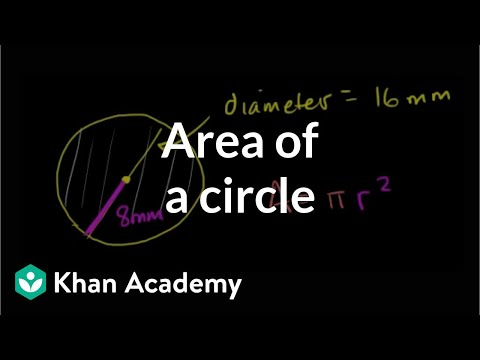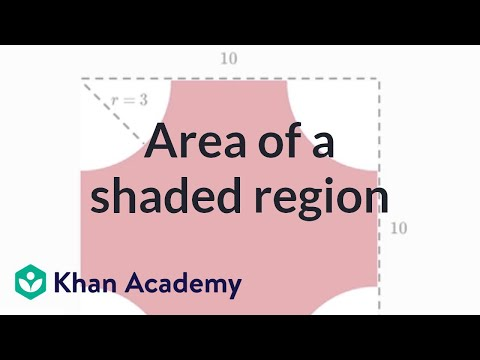Video

# Partial circle area and arc length (Full video)

Description: Sal finds the area of a semicircle and the arc length of a partial circle. We know that the area of a circle is equal to pi times our radius squared. So, if we think about the entire circle, what is the area going to be? Our radius is equal to two, so the area, if we're talking about the whole circle, it would be equal to pi times two squared.

### Other videos you might be interested in### Area of a circle (Full video)